# 13.1 Sequences and their notations  (Page 7/15)

 Page 7 / 15

$\left(\frac{12}{6}\right)!$

$\frac{12!}{6!}$

$665,280$

$\frac{100!}{99!}$

For the following exercises, write the first four terms of the sequence.

${a}_{n}=\frac{n!}{{n}^{\text{2}}}$

First four terms: $1,\frac{1}{2},\frac{2}{3},\frac{3}{2}$

${a}_{n}=\frac{3\cdot n!}{4\cdot n!}$

${a}_{n}=\frac{n!}{{n}^{2}-n-1}$

First four terms: $-1,2,\frac{6}{5},\frac{24}{11}$

${a}_{n}=\frac{100\cdot n}{n\left(n-1\right)!}$

## Graphical

For the following exercises, graph the first five terms of the indicated sequence

${a}_{n}=\frac{{\left(-1\right)}^{n}}{n}+n$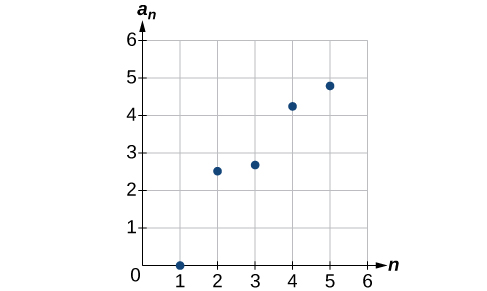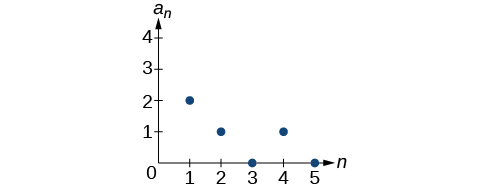${a}_{n}=\frac{\left(n+1\right)!}{\left(n-1\right)!}$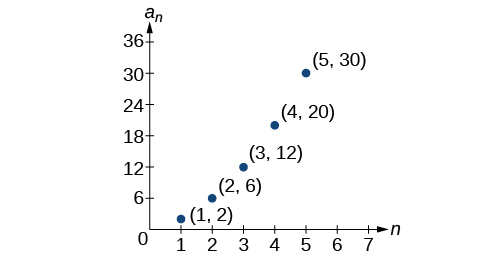For the following exercises, write an explicit formula for the sequence using the first five points shown on the graph.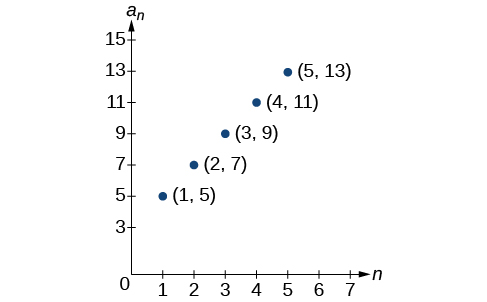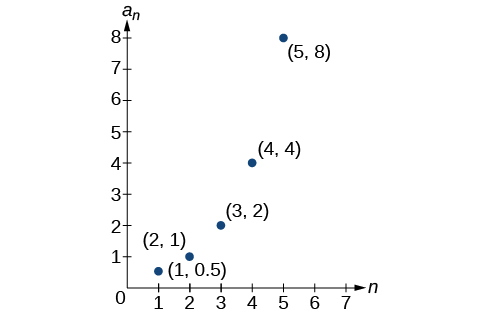${a}_{n}={2}^{n-2}$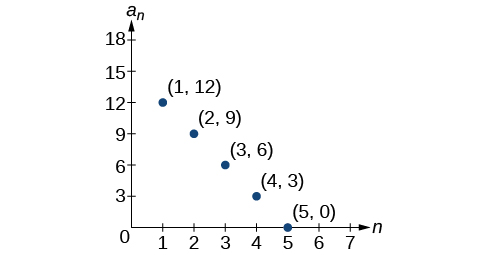For the following exercises, write a recursive formula for the sequence using the first five points shown on the graph.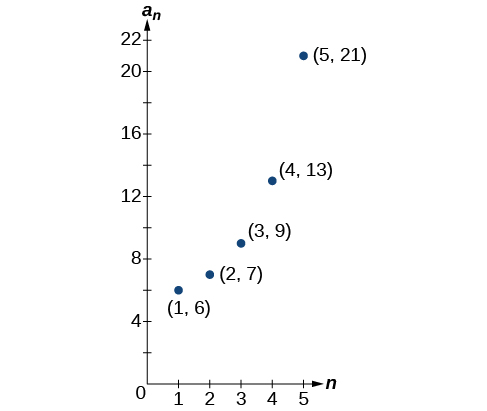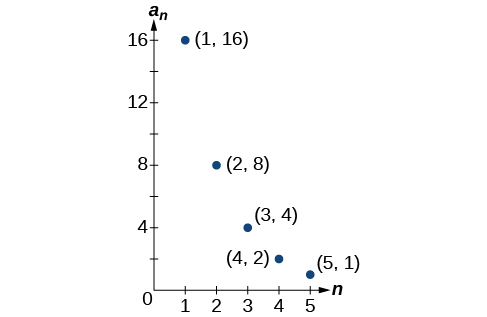## Technology

Follow these steps to evaluate a sequence defined recursively using a graphing calculator:

• On the home screen, key in the value for the initial term $\text{\hspace{0.17em}}{a}_{1}\text{\hspace{0.17em}}$ and press [ENTER] .
• Enter the recursive formula by keying in all numerical values given in the formula, along with the key strokes [2ND] ANS for the previous term $\text{\hspace{0.17em}}{a}_{n-1}.\text{\hspace{0.17em}}$ Press [ENTER] .
• Continue pressing [ENTER] to calculate the values for each successive term.

For the following exercises, use the steps above to find the indicated term or terms for the sequence.

Find the first five terms of the sequence Use the> Frac feature to give fractional results.

First five terms: $\frac{29}{37},\frac{152}{111},\frac{716}{333},\frac{3188}{999},\frac{13724}{2997}$

Find the 15 th term of the sequence

Find the first five terms of the sequence

First five terms: $2,3,5,17,65537$

Find the first ten terms of the sequence

Find the tenth term of the sequence

${a}_{10}=7,257,600$

Follow these steps to evaluate a finite sequence defined by an explicit formula. Using a TI-84, do the following.

• In the home screen, press [2ND] LIST .
• Scroll over to OPS and choose “seq(” from the dropdown list. Press [ENTER] .
• In the line headed “Expr:” type in the explicit formula, using the $\text{\hspace{0.17em}}\left[\text{X,T},\theta ,n\right]\text{\hspace{0.17em}}$ button for $\text{\hspace{0.17em}}n$
• In the line headed “Variable:” type in the variable used on the previous step.
• In the line headed “start:” key in the value of $\text{\hspace{0.17em}}n\text{\hspace{0.17em}}$ that begins the sequence.
• In the line headed “end:” key in the value of $\text{\hspace{0.17em}}n\text{\hspace{0.17em}}$ that ends the sequence.
• Press [ENTER] 3 times to return to the home screen. You will see the sequence syntax on the screen. Press [ENTER] to see the list of terms for the finite sequence defined. Use the right arrow key to scroll through the list of terms.

Using a TI-83, do the following.

• In the home screen, press [2ND] LIST .
• Scroll over to OPS and choose “seq(” from the dropdown list. Press [ENTER] .
• Enter the items in the order “Expr” , “Variable” , “start” , “end” separated by commas. See the instructions above for the description of each item.
• Press [ENTER] to see the list of terms for the finite sequence defined. Use the right arrow key to scroll through the list of terms.

For the following exercises, use the steps above to find the indicated terms for the sequence. Round to the nearest thousandth when necessary.

List the first five terms of the sequence ${a}_{n}=-\frac{28}{9}n+\frac{5}{3}.$

List the first six terms of the sequence

First six terms: $0.042,0.146,0.875,2.385,4.708$

List the first five terms of the sequence ${a}_{n}=\frac{15n\cdot {\left(-2\right)}^{n-1}}{47}$

List the first four terms of the sequence ${a}_{n}={5.7}^{n}+0.275\left(n-1\right)!$

First four terms: $5.975,32.765,185.743,1057.25,6023.521$

List the first six terms of the sequence ${a}_{n}=\frac{n!}{n}.$

## Extensions

Consider the sequence defined by ${a}_{n}=-6-8n.$ Is ${a}_{n}=-421$ a term in the sequence? Verify the result.

If $\text{\hspace{0.17em}}{a}_{n}=-421\text{\hspace{0.17em}}$ is a term in the sequence, then solving the equation $-421=-6-8n$ for $n$ will yield a non-negative integer. However, if $\text{\hspace{0.17em}}-421=-6-8n,\text{\hspace{0.17em}}$ then $n=51.875$ so ${a}_{n}=-421$ is not a term in the sequence.

What term in the sequence ${a}_{n}=\frac{{n}^{2}+4n+4}{2\left(n+2\right)}$ has the value $41?$ Verify the result.

Find a recursive formula for the sequence ( Hint : find a pattern for $\text{\hspace{0.17em}}{a}_{n}\text{\hspace{0.17em}}$ based on the first two terms.)

${a}_{1}=1,{a}_{2}=0,{a}_{n}={a}_{n-1}-{a}_{n-2}$

Calculate the first eight terms of the sequences ${a}_{n}=\frac{\left(n+2\right)!}{\left(n-1\right)!}$ and ${b}_{n}={n}^{3}+3{n}^{2}+2n,$ and then make a conjecture about the relationship between these two sequences.

Prove the conjecture made in the preceding exercise.

$\frac{\left(n+2\right)!}{\left(n-1\right)!}=\frac{\left(n+2\right)·\left(n+1\right)·\left(n\right)·\left(n-1\right)·...·3·2·1}{\left(n-1\right)·...·3·2·1}=n\left(n+1\right)\left(n+2\right)={n}^{3}+3{n}^{2}+2n$

answer and questions in exercise 11.2 sums
how do u calculate inequality of irrational number?
Alaba
give me an example
Chris
and I will walk you through it
Chris
cos (-z)= cos z .
what is a algebra
(x+x)3=?
what is the identity of 1-cos²5x equal to?
__john __05
Kishu
Hi
Abdel
hi
Ye
hi
Nokwanda
C'est comment
Abdel
Hi
Amanda
hello
SORIE
Hiiii
Chinni
hello
Ranjay
hi
ANSHU
hiiii
Chinni
h r u friends
Chinni
yes
Hassan
so is their any Genius in mathematics here let chat guys and get to know each other's
SORIE
I speak French
Abdel
okay no problem since we gather here and get to know each other
SORIE
hi im stupid at math and just wanna join here
Yaona
lol nahhh none of us here are stupid it's just that we have Fast, Medium, and slow learner bro but we all going to work things out together
SORIE
it's 12
what is the function of sine with respect of cosine , graphically
tangent bruh
Steve
cosx.cos2x.cos4x.cos8x
sinx sin2x is linearly dependent
what is a reciprocal
The reciprocal of a number is 1 divided by a number. eg the reciprocal of 10 is 1/10 which is 0.1
Shemmy
Reciprocal is a pair of numbers that, when multiplied together, equal to 1. Example; the reciprocal of 3 is ⅓, because 3 multiplied by ⅓ is equal to 1
Jeza
each term in a sequence below is five times the previous term what is the eighth term in the sequence
I don't understand how radicals works pls
How look for the general solution of a trig function
stock therom F=(x2+y2) i-2xy J jaha x=a y=o y=b
sinx sin2x is linearly dependent
cr
root under 3-root under 2 by 5 y square
The sum of the first n terms of a certain series is 2^n-1, Show that , this series is Geometric and Find the formula of the n^th
cosA\1+sinA=secA-tanA
Wrong question
why two x + seven is equal to nineteen.
The numbers cannot be combined with the x
Othman
2x + 7 =19
humberto
2x +7=19. 2x=19 - 7 2x=12 x=6
Yvonne
because x is 6
SAIDIByBy Stephen VoronBy Sean WiffleBoyBy Nicole BartelsBy JavaChamp TeamBy Yasser IbrahimBy DanielrosenbergerBy Robert MurphyBy P. Wynn NormanBy Marriyam RanaBy John Gabrieli